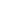科研动态最新资讯

• 天元讲堂（10.13，10.14）​李抒行报告（共两讲）
• 浏览量：165 发布人： 发布时间：2019-10-08
• 报告题目Construction and Nonexistence of Strong External Difference Families

报告人：李抒行 马格德堡大学（Otto von Guericke University Magdeburg

报告时间20191013日，15:30-16:30

报告地点：精正楼301

报告摘要Strong external difference family (SEDF) is an emerging combinatorial configuration motivated by cryptography applications. It contains a collection of subsets with equal sizes in a finite group,so that the differences generated from these subsets cover each non-identity elements of the group the same number of times. In this talk, we give an overview of SEDFs in finite abelian groups, with an emphasis on those having more than two subsets.We present an elegant construction of a (243,11,22,20)-SEDF in $\mathbb{C_3^5}$, which involves various mathematical objects including partial difference sets, projective geometry and sporadic finite simple group, and is indeed the only known example of SEDF having more than two subsets. Moreover, employing character theory, we derive a series of nonexistence results, which indicates the rareness of such SEDFs. Our nonexistence results culminates in the following theorem: there exists no strong external difference family with more than two subsets in abelian group $G$ of order $v$, where $v$ is a product of at most three not necessarily distinct primes, except possibly when $G=C_p^3$ and $p$ is a prime greater than $3 \times 10^{12}$.

This is joint work with Jonathan Jedwab, Ka Hin Leung and Theo Fanuela Prabowo.

报告题目Formal Duality in Finite Abelian Groups

报告人：李抒行 马格德堡大学（Otto von Guericke University Magdeburg

报告时间20191014日，9:00-10:00

报告地点：精正楼301

报告摘要In Euclidean space, a periodic configuration is a union of finitely many translations of a lattice. In particular, energy-minimizing periodic configurations are those which possess minimum energy. Finding energy-minimizing periodic configurations is an interesting problem, not only because of its theoretical significance in physics, but also its connection with the famous sphere packing problem. On the other hand, the search of energy-minimizing periodic configurations is notoriously difficult and very few theoretical results are known. Nevertheless, an insightful idea due to Cohn, Kumar, Reiher and Schurmann, enables us to study the energy-minimizing periodic configurations from a combinatorial viewpoint. Roughly speaking, among pairs of energy-minimizing periodic configurations, they revealed a remarkable symmetry named formal duality. Furthermore, they translated the formal duality into a purely combinatorial context, where the corresponding configuration was called a formally dual pair, which is a pair of subsets in a finite abelian group satisfying a difference-set-like property. In this talk, we will give an overview and present some recent results of formally dual pairs, which involve constructions, nonexistence results and characterizations of formally dual paris.

This is joint work with Alexander Pott and Robert Schuller.

欢迎参加！